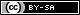# Dev Blog

 ./dev Original theme by orderedlist (CC-BY-SA) Where applicable, all content is licensed under a CC-BY-SA.## Kelly Criterion

The Wikipedia article on the Kelly criterion might say more, but here is a simple derivation.

Assuming you have a coin with probability $p$ of coming up heads and odds of $b:1$, the Kelly criterion states:

$$f^* = \frac{bp-q}{b}$$

Where $f^*$ is the fraction of your money pot the Kelly criterion tells you to bet and $q=1-p$.

That is:

$$f^* = \frac{bp-(1-p)}{b} \ = \frac{p(b+1)-1}{b}$$

Assuming the strategy is to bet a fraction of your bank roll every round, with $W_0$ as the initial bank roll, $n$ time units and $W_n$ as your winnings at time $n$:

$$W_n = (1 + br)^{pn} (1 - r)^{(1-p)n} W_0$$

Taking logarithms, setting the derivative with respect to $r$ and solving:

$$\begin{array} . & \frac{d}{dr} \ln(W_n) &= \frac{d}{dr} ( pn \ln(1+br) + (1-p)n \ln(1-r) + \ln(W_0) ) \\ \to & 0 &= \frac{pnb}{1+br} - \frac{(1-p)n}{1-r} \\ \to & \frac{(1-p)n}{1-r} &= \frac{pnb}{1+br} \\ \to & \frac{1-p}{1-r} &= \frac{pb}{1+br} \\ \to & (1-p) (1+br) &= pb (1-r) \\ \to & 1 - p + br - pbr &= pb - pbr \\ \to & 1 - p + br &= pb \\ \to & r &= \frac{pb + p - 1}{b} \\ \to & r &= \frac{b(p + 1) - 1}{b} \\ \end{array}$$